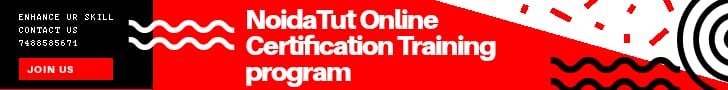FREE E LEARNING PLATFORM

# Find number of Digits and White Spaces in a StringThis program takes the input string (entered by user) and counts the number of digits and white spaces in the String.

### Example: Program to count number of digits and white spaces in a String

In this program we have a string object to store the input string. If you want you can also use char array in place of string object but the problem with char array is that it has a fixed size, which means if you define a char array of size 100 and user enters string longer than the program will truncate the string.

Here we are using for loop to traverse the string from starting character till end char (‘\0’) and counting the number of digits and white spaces.

```#include <iostream>
using namespace std;
int main(){
string str;
int digitCounter = 0, spaceCounter = 0;
cout << "Enter any string: ";
getline(cin, str);
//'\0 represent end of string
for(int i = 0; str[i]!='\0'; i++) {
if(str[i]>='0' && str[i]<='9') {
digitCounter++;
}
else if(str[i]==' ') {
spaceCounter++;
}    }
cout << "Digits in String: " << digitCounter << endl;
cout << "White Spaces in String: " << spaceCounter << endl;
return 0;
}```

### Output

```Enter any string: My Roll No is: 1099234
Digits in String: 7
White Spaces in String: 4```